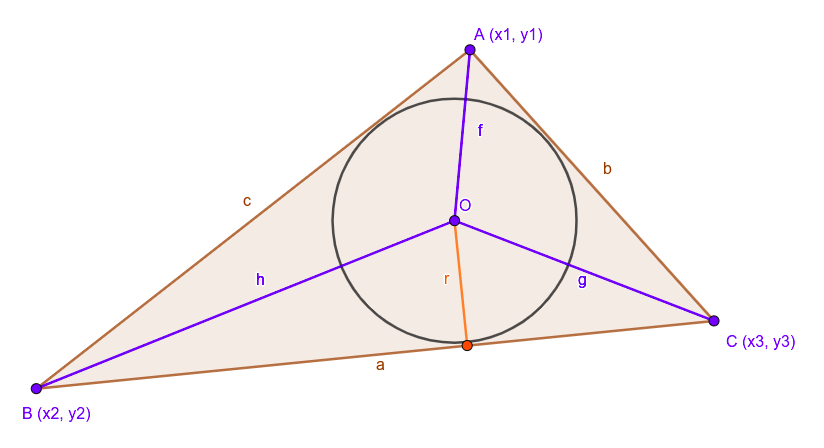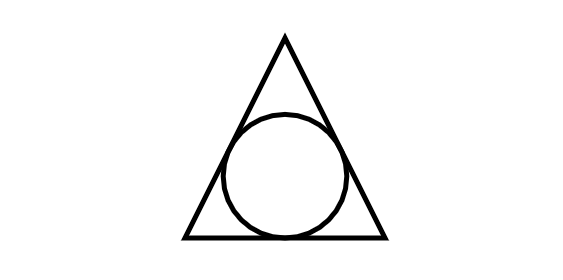Related Articles
How to draw Inscribed circle of a triangle using p5.js ?
• Last Updated : 29 Jan, 2020

In this article, we will see how to draw inscribed circle (incircle) form a triangle. The incircle is nothing but the largest possible circle that can be drawn on the inside of a plane figure. So basically all sides (three) of triangle must be touched by the circle surface but not to cross the sidebars.

Mathematics Background: In given triangle ABC as shown below.• The center of the inscribed-circle (known as in-center) of a triangle is defined as:Implementation:

• Step 1: In Computer Graphics we represent a triangle by its angular points rather than its side length meaning the input would be the vertices of the triangle! So we need to convert that into the side-lengths a, b, c so that we could use it for the formula. To achieve this we use this simple getSides helper function:
Code:
 // Get Sides from Angular pointsfunction getSides(Ax, Ay, Bx, By, Cx, Cy){  return {    a: dist(Bx, By, Cx, Cy),    b: dist(Cx, Cy, Ax, Ay),    c: dist(Ax, Ay, Bx, By),  }}
• Step 2: Now, we can convert angular points to sides we can simply plug the formula to get the desired result. Here, the full P5.js sketch which draws the incircle of a triangle:

Code:

 function setup() {  createCanvas(640, 480);}  function draw() {  background(255);  noFill()  stroke(0)  strokeWeight(5)  triangle(320, 140, 220, 340, 420, 340)  drawInCircle(320, 140, 220, 340, 420, 340)  noLoop()}  function drawInCircle(x1, y1, x2, y2, x3, y3){  let side=getSides(x1, y1, x2, y2, x3, y3)  let a=side.a, b=side.b, c=side.c;  let inCenter=getIncenter(a, b, c, x1, y1, x2, y2, x3, y3);  let inRadius=getInradius(a, b, c);  circle(inCenter.x, inCenter.y, 2*inRadius)}  // Helper Function: Get Sides from Angular pointsfunction getSides(Ax, Ay, Bx, By, Cx, Cy){  return {    a: dist(Bx, By, Cx, Cy),    b: dist(Cx, Cy, Ax, Ay),    c: dist(Ax, Ay, Bx, By),  }}function getIncenter(a, b, c, x1, y1, x2, y2, x3, y3){  return {    x: (a*x1 + b*x2 + c*x3)/(a + b + c),    y: (a*y1 + b*y2 + c*y3)/(a + b + c)  }}  function getInradius(a, b, c){  let s=(a+b+c)/2    // Semi-perimeter  let area=sqrt(s*(s-a)*(s-b)*(s-c))  return area/s}
• Output:Note: You can edit and run your code by using online editor.

My Personal Notes arrow_drop_up